Metre Bridge-Resistance of a wire

# Our Objective:

To find the resistance of a given wire using a metre bridge and hence determine the specific resistance of its materials.

# Theory

## Wheatstone’s principleThe metre bridge is operates under Wheatstone’s principle.  Here, four resistors P, Q, R, and S are connected to form the network ABCD. The terminals A and C are connected to a battery, and the terminals C and D are connected to a galvanometer through keys K1 and K2 respectively.                                                                                                                                                   In the balancing condition, there is no deflection on the galvanometer. Then,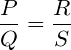## Metre Bridge apparatus

The metre bridge, also known as the slide wire bridge consists of a one metre long wire of uniform cross sectional area, fixed on a wooden block. A scale is attached to the block. Two gaps are formed on it by using thick metal strips in order to make the Wheat stone’s bridge. The terminal B between the gaps is used to connect galvanometer and jockey.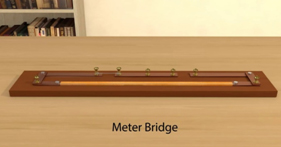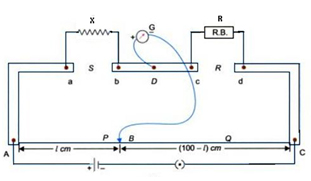A resistance wire is introduced in gap S and the resistance box is in gap R. One end of the galvanometer is connected to terminal D and its other end is connected to a jockey. As the jockey slides over the wire AC, it shows zero deflection at the balancing point (null point).

If the length AB is, then the length BC is  ( 100-).

Then, according to Wheatstone’s principle;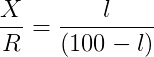Now, the unknown resistance can be calculated as,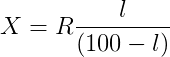The specific resistance or resistivity of the material of the wire can be then calculated by using the relation,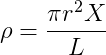; Where L be the length of the wire and r be its radius.

# Learning Outcomes:

• Students understand Wheatstone’s bridge and Wheatstone’s principle.
• Students verify Wheatstone’s principle.
• Students correlate the principle of Wheatstone’s bridge with metre bridge experiment.

Cite this Simulator: